Features

# Curved Surfaces Using Bézier Patches

Traditionally, 3D geometry used in games has been stored as static polygons in a structure like a mesh or BSP tree. However, by taking advantage of today's powerful graphics hardware and making use of Bezier patches, games can realize more organic shapes in real-time.

Until recently, 3D games have had a fairly strict limitation on the number of triangles that could be displayed per second. This limited the amount of visual realism that could be attained. With the advent of faster CPUs and powerful consumer level 3D video cards, more complex and realistic geometry can now be created. One of the more dramatic ways of increasing visual realism is by implementing curved surfaces. One way to create curved surfaces is by using Bézier patches, a procedural geometry technique. Figure 1, a screenshot taken from id Software's Quake III: Arena, shows what sort of effect on architecture using these curves can have. Other upcoming games, such as Shiny Entertainment's Messiah, also incorporate curved surfaces to give them a more realistic and organic look. There's pretty good motivation to learn how they do it.

Procedural Geometry

Traditionally, geometry used for computer games has been stored as static polygons in some structure such as a mesh or BSP tree. Every polygon is defined during design and used later during display. One way to take advantage of the increased 3D horsepower available in new computers would be simply to use smaller triangles and more of them when defining our static geometry. There are several drawbacks to this approach:

• Large storage requirements. As more detail is desired, the numbers of triangles required will take up more memory and disk space and take longer to traverse.
• Lack of scalability. The amount of geometry defined would be created for the best case machine. To display the geometry with a reasonable frame rate on a less than optimal machine would require storing multiple models with decreasing detail or implementing a real-time polygon reduction algorithm. There is still the problem that future, more capable machines will be stuck with the static geometry designed for older systems.
• Inability to naturally describe shapes like curves. The only way to represent curved surfaces with triangles is by using a large number of triangles to approximate the surface.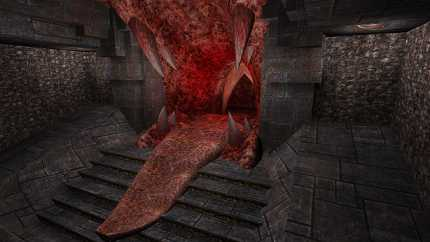Figure 1: Quake 3 Arena uses Bézier patches to beautifully render this demonic tongue.

In my mind, scalability is going to be one of the largest issues facing game developers in the future. There is going to be a large installed base of Pentium-class machines with Voodoo cards that you do not want to leave out, but at the same time you would like your game to run great on someone's shiny new Pentium III with a SLI Voodoo2 setup. Currently, most games are designed for a minimum system in mind with faster systems just attaining astronomically high frame rates that monitors can not support. It would be nice for the increased horsepower to go towards better visual quality, not just a higher frame rate.

An alternative to the traditional static polygon list that addresses many of the problems listed above is to use procedural techniques to generate the polygons. Procedural geometry involves taking some parameters and following a set procedure to generate an arbitrary number of vertices or polygons. Procedural geometry addresses the deficiencies of static geometry listed above:

• It's compact. Just a few parameters can generate any number of intermediate values.
• It's scalable. The number of vertices or polygons generated can be adjusted to allow the game to target a specific frame rate. The faster the machine, the more triangles that can be generated.
• It can directly describe curves.

It is important to keep in mind that while ultimately triangles are used with both procedural and static forms, the procedural form generates them at runtime to a desired degree of fineness, whereas with static geometry, they are defined at design time to a predetermined degree of detail.

On Lines

One of the simplest procedural geometry forms that everyone should be familiar with are lines. Given the two end points of a line segment, any desired number of intermediate points can be generated using the line equation. It is possible to store a large number of points that are located on the line instead, but we all know that would be silly. However, it does illustrate some of the problems of static geometry. Instead of storing just two points, a large number of points would need to be stored. Also, these points would be targeted for a specific resolution. If the resolution is reduced, redundant points exist, and if the resolution is increased, gaps in the line will appear. It is apparent that the procedural form is preferred.

Let's examine lines to help us develop a notation that will be useful to us later. The equation below shows the parametric form of the line equation.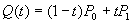t is the parameterized value ranging between 0 and 1 inclusive and P0 and P1 are the line's endpoints. When t is 0, the equation returns P0 and when t is 1, the equation returns P1. This equation can be rewritten as follows.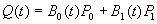Where: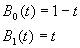We will call B0(t)and B1(t) the basis functions of the parametric line equation. They are sometimes also called blending functions, because they define how the two endpoints are blended to get the value for the line at t. The line equation can be further rewritten compactly as: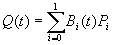This is the analytical version of the parametric line equation. Most descriptions of parametric curves will be presented using this form. Yes, lines are curves, just very straight ones.

Developing Bézier Curves

How might we come up with a parametric form for a curve using notation similar to above? We would like to describe a curve that passes through its endpoints and curves towards a third point. Figure 2 shows a possible setup where P0 and P2 are the endpoints of the curve and P1 is the point to approach. A line segment can be defined where one endpoint, Pa(t), is interpolated from P0 to P1 and its other endpoint, Pb(t), is interpolated from P1 to P2. This is shown in Figure 3. The desired point on the curve can then be found by interpolating between Pa(t) and Pb(t). This formulation is developed in the equations below.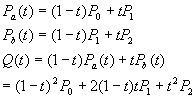Figure 4 shows the curve that is generated when t goes from 0 to 1.

Using the notation we developed for the line, this becomes: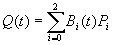Where B0, B1, and B2 are the basis functions shown below: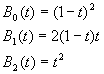Some features to note about this curve formulation: the curve passes through the endpoints, the line segment from P0 to P1 is tangent to the curve at P0, and the line segment from P1 to P2 is tangent to the curve at P2. This leads to a very intuitive description of a curve and is easy for artists to manipulate. Another aspect of this curve is that it is contained in the convex hull of its control points. The convex hull can be thought of as the polygon that would be formed by stretching a rubber band around the control points. This property can be used for rough hit detection or similar things.

Well, what we have done is develop the definition of a quadratic Bézier curve. This geometric interpretation of the Bézier curve is attributed to de Casteljau. It can be thought of as a generalization of the parametric line equation. If we would like to develop a Bézier curve of a higher degree, the process used above of interpolating new line segments can be applied recursively. When this is done, the basis functions can be defined as follows, where n is the degree and i is which basis function from 0 to n :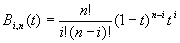These are known as the Bernstein polynomials. If n is 2, this generates the basis functions for the quadratic Bézier curve that we discovered earlier. Interestingly, when n is 1, this generates the blending functions for the parametric line equation, further reinforcing that the Bézier curve can be thought of as the generalization of a line to higher orders. Typically, quadratic (n = 2) or cubic (n = 3) curves are used. Quadratic curves are easier to calculate and require fewer control points while cubic curves allow for a wider variety of curves at a higher computational cost. Figure 5 shows an example of each type of curve. For the rest of this article, I am going to restrict the discussion to the quadratic forms, but everything can be extended to higher degree curves, though cubic is the highest order ever used in practice.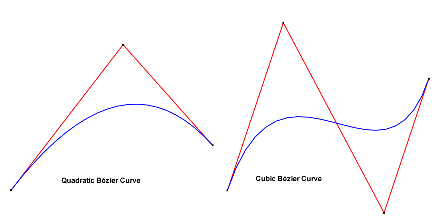Figure 5: Quadratic and cubic curves. Quadratics are easier to calculate (i.e. faster to render).

Connect the Curves

Usually one curve is not enough to describe what you want. Because Bézier curves pass through their end control points, a continuous sequence of curves can be created by having them share endpoints. The curves will just physically connect, but not necessarily smoothly. This is called geometric continuity of order 0, often referred to as G0. Often it is desirable to have the curves appear to connect smoothly. This is referred to as geometric continuity of order 1, or G1. To have the curves connect smoothly, the tangent lines to the two curves at the shared point should have the same slope. This can be guaranteed by having the shared endpoint and the adjacent control points be collinear.

Geometric continuity addresses how the curve appears, but there is more rigorous form of continuity, known as analytical continuity, that addresses how the curve behaves as well as appears. This type of continuity is defined in terms of derivatives and is represented by C instead of G in discussions. In general, C0 is equal to G0. C1 means that the first derivatives of the two curves are equal at the shared point. G1 is equal to C1 when the tangent lines not only have the same slope, but the same magnitude as well. The first derivative is often interpreted as the velocity, so it is important for a curve to have C1 continuity when the curve is used to represent a path that a camera might take or else there will be a noticeable jump in the velocity as the camera passes through the shared point. If the second derivatives match, implying C2 continuity, the acceleration through the shared point matches as well.

For modelers, maintaining geometric continuity is usually sufficient, but often the more strict analytical continuity will be required when describing paths.

Some Uses for Curves

We examined Bézier curves as a step along our path to using them to generate curved surfaces, but they do have some uses of their own. Primary among these uses is to have them define paths for things like cameras and entities. Now your creatures will not have to move only along straight lines, but can move along curves as well.

Rendering Bézier Curves

Now that we have defined Bézier curves, we would like to display them. There are a couple of ways to do this. The most straightforward way, shown in Listing 1, is to evaluate the curve equation along constant steps in t between 0 and 1. The finer the steps that are taken, the higher quality the curve will be. Since the basis functions are polynomials, this process can be sped up by using forward differencing. Figure 6 shows the same curve rendered with different step sizes.

Listing 1.

/*

* Calculates a Bezier curve directly by iterating along the curve

* for the desired number of steps.

*

* Point is an object that has the x, y, and z coordinates and defines

* some mathematical operations for points. See the project accompanying

* the article for its definition.

*

* G is the geometry vector, the three control points for the curves.

* steps is the number of steps to take along the curve.

*/

void QuadraticBezierIterate(Point G[], int steps) {

float stepSize = 1.0 / steps;

float t = stepSize;

// Draw the curve as a line strip

glBegin(GL_LINE_STRIP);

// First control point is on the curve

glVertex3f(G.x, G.y, G.z);

// Calculate intermediate points

for(int step = 1; step < steps; step++, t += stepSize) {

// Calculate the blending functions

float b0 = (1 - t) * (1 - t);

float b1 = 2.0 * t * (1 - t);

float b2 = t * t;

// Blend

float x = b0 * G.x + b1 * G.x + b2 * G.x;

float y = b0 * G.y + b1 * G.y + b2 * G.y;

float z = b0 * G.z + b1 * G.z + b2 * G.z;

// Emit a vertex

glVertex3f(x, y, z);

}

// Last control point is on the curve

glVertex3f(G.x, G.y, G.z);

glEnd();

}

Divide and Conquer

There is another way to calculate points on the curve known as subdivision. This form is come about by reparameterizing the curve into a left half and a right half. This generates two sets of control points, one for each half of the original curve. The endpoints of these new curves, which are represented in the new control points, are on the curve, so we will have new points on the curve. This process can be applied recursively to generate as many points on the curve as desired.

The left half of the curve is developed below using the matrix formulation. The matrix form of the quadratic Bézier curve is shown below.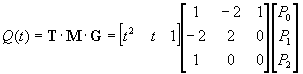Reparameterizing this curve to go from 0 to ½ becomes: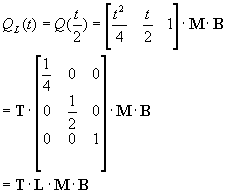We can further manipulate this equation to generate a matrix that when multiplied by the geometry vector of the original control points will create new control points that define a Bézier curve for the first half of the original curve as follows: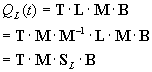After some matrix calculations, SL is found to be: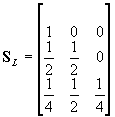Similarly, the curve can be reparameterized to find the matrix that will generate the new control points for a Bézier curve that duplicates the right half of the original curve. When this is done, a SR is found to be:There are several advantages to this approach. To generate new points, only the matrix multiplies are needed, t does not need to be used at all. The subdivision matrices involve only division by powers of two, which can be pretty efficient, particularly if fixed point representations are being used, since then it only involves a shift.

A curve can be generated by recursively subdividing the control points and using the newly generated endpoints as points on the curve. It would also be possible to use the middle control point as a point on the curve, though technically it is not, but as the subdivision gets further and further refined, this point gets closer and closer to the curve. The subdivision can either be set to only go to a certain depth, or it can be designed to stop when the segment is deemed flat enough. Listing 2 uses recursive subdivision to draw a Bézier curve. A little speed can be gained if the code is recrafted to take advantage of the fact that the two new halves of the subdivided curve share the middle endpoint.

Listing 2

/*

* Calculates a Bezier curve using subdivision to the desired depth.

*

* Point is an object that has the x, y, and z coordinates and defines

* some mathematical operations for points. See the project accompanying

* the article for its definition.

*

* G is the geometry vector, the three control points for the curves.

* level is the depth to subdivide.

*/

void QuadraticBezierSubdivide(Point G[], int level) {

// Draw a line if level 0 using the end points

if(level == 0) {

glBegin(GL_LINES);

glVertex3f(G.x, G.y, G.z);

glVertex3f(G.x, G.y, G.z);

glEnd();

return;

}

// New geometry vectors for the left and right halves of the curve

Point Gl;

Point Gr;

// Subdivide

Gl = G;

Gl = (G + G) * 0.5f;

Gr = (G + G) * 0.5f;

Gl = Gr = (Gl + Gr) * 0.5f;

Gr = G;

// Call recursively for left and right halves

}

Bézier Patches

A question arises, what would happen if the control points used to create a curve were actually on curves themselves? In other words, what if the curve equation was dependant on two variables instead of one and looked like the one below shown for quadratic Bézier curves.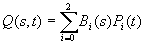Now, if Pi(t) is itself defined as a quadratic Bézier curve, we end up with the following.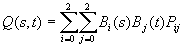Bi(s) and Bj(t) are the usual quadratic Bézier blending functions and Pij are nine control points in the form of a three by three grid, since each of the three control points for the curve are defined along a curve themselves that requires three control points. This is known as the biquadratic formulation for a Bézier patch and we can generate a curved surface by varying s and t between 0 and 1. Figure 7 shows a biquadratic patch with its nine control points. It can easily be extended to use cubic Bézier curves, bicubic Bézier patches, which would require sixteen control points. As the order of the base curve increases, the number of required control points will increase by the square of the order, so often for the sake of speed it is best to use lower order curves.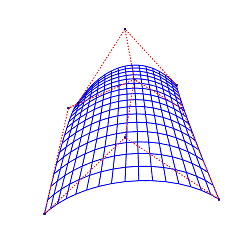Figure 7: A biquadratic patch with its nine control points. [zoom]

From examining the equation for the surface, it is pretty clear that setting t to a constant value will generate a set of control points and then varying s will create a Bézier curve using those control points that is on the surface. The process is symmetrical, so s could be set to a constant value and t varied to generate curves on the surface going in the other direction. This leads to some useful properties to know about Bézier patches.

• The edges are Bézier curves defined by just the control points along the edge. This is true because at the edges, s or t is equal to either 0 or 1 depending on the which of the four edges, and the blending functions when that is the case just return the control points defined along the appropriate edge.
• As a result of the first, the patches pass through the control points at their corners. This is true because the edges are the Bézier curves defined by the control points on the edge, and Bézier curves pass through their end control points.
• Bézier patches are contained within the convex hull of their control points, which is useful for coarse collision detection. The convex hull of the control points can be thought of as the solid you would get if you were to stretch a rubber sheet around the control points.

I know that last paragraph is confusing. I recommend playing with the equation for the surface by alternately setting s to 0 and 1 and seeing what control points result and then do the same for t. With a little effort, the properties of Bézier patches described should become clear.

Making a Quilt

Bézier patches can be connected in much the same way that Bézier curves are. If the patches share the same control points along an edge, they will join at the Bézier curve defined by those control points. The patches will just be G0 continuous, and a crease may be visible. If you want more smoothness, you will need to be careful to arrange it so that all of the control points are G1 continuous along the edge. If the patch is to be joined with a regular polygon, the control points along the edge to be joined should be collinear, since that will make the patch flat at that edge.

A set of patches can ultimately be thought of being connected in three ways, shown in Figure 8:

• Planar. The set of patches never connect back up with themselves. This is the normal case.
• Cylindrical. The last patch edge wraps around and connects back up to the leading edge, forming a cylindrical shape. This is useful for making limbs, and so on.
• Toroidal. If all edges wrap back on themselves, a donut shape is formed, known as a torus. This is probably the least common configuration.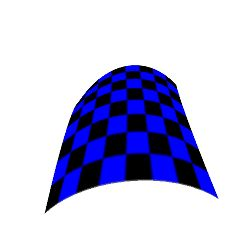Figure 8: A planar set of Bézier patches

Rendering Bézier Patches

Generating the vertices for a patch is not too difficult now that we know how to generate them for a curve. Generally what is done is to generate points of constant s at equal steps of t. The symmetrical operation of curves of constant t at equal steps of s could also be done. The same number of steps are taken along s and t. These steps along s and t could be calculated directly or through subdivision. What we end up with is a rectangular grid of points on the patch. We could simply render these points as a quadrilaterals, but a problem arises: there is no guarantee that the quadrilateral is flat. This may be a problem for some renderers. For most purposes, it is easiest to render using triangles, since the slight inaccuracies of not being on the ideal flat surface are negligible and are more than made up for with speed. Listing 3 renders a patch using triangle strips.

The decision as to how finely to tessellate the surface is up to the developer. It is usually based on the distance to the model and the available power of the machine doing the rendering.

Listing 3

/*

* This fragment takes the points returned by a function that subdivides a

* Bezier patch and renders them using triangle strips.

*

* Point is an object that has the x, y, and z coordinates and defines

* some mathematical operations for points. See the project accompanying

* the article for its definition.

*

* LevelToWidth[] is a lookup table that calculates the widths of rows given

* the depth of recursion.

*

* cpoints is a 3 x 3 matrix of control points for the quadratic Bezier patch.

*/

int width = LevelToWidth;

// For all the strips

for(int i = 0; i < width - 1; i++) {

// Emit the vertices on the strip

glBegin(GL_TRIANGLE_STRIP);

for(int j = 0; j < width; j++) {

glVertex3f(p[i * width + j].x, p[i * width + j].y, p[i * width + j].z);

glVertex3f(p[(i + 1) * width + j].x, p[(i + 1) * width + j].y, p[(i + 1) * width + j].z);

}

glEnd();

}

// Free the points

delete[] p;

Right now, we have naked patches and would like to put some clothing on them. Applying a texture map to a patch is pretty straightforward. The simplest way is to parameterize the u and v coordinates for the texture using the s and t used to create the vertices. Listing 4 renders a texture mapped Bézier patch using this method. Constant steps along the parameter do not guarantee constant steps in arclength on the curve. This can lead to irregular texture stretching. This effect can be minimized by have the control points by fairly evenly spaced. To actually generate the texture coordinates using constant steps in arclength is pretty compute intensive and beyond the scope of this article, but it is possible.

Another feature of Bézier patches is the ability to dynamically modify the control points. This can simulate ripples in water, flags blowing in the breeze, bouncing body parts, and so on, while only manipulating a few control points.

Listing 4

/*

* This fragment takes the points returned by a function that subdivides a

* Bezier patch and renders them using triangle strips. Additionally, the

* triangles are texture mapped assuming the active texture maps directly to

* the patch.

*

* Point is an object that has the x, y, and z coordinates and defines

* some mathematical operations for points. See the project accompanying

* the article for its definition.

*

* LevelToWidth[] is a lookup table that calculates the widths of rows given

* the depth of recursion.

*

* cpoints is a 3 x 3 matrix of control points for the quadratic Bezier patch.

*/

glColor3f(0.0, 0.0, 1.0);

glEnable(GL_TEXTURE_2D);

int width = LevelToWidth;

// Step size along texture map

float step = 1.0 / (width - 1);

// For all the strips

for(int i = 0; i < width - 1; i++) {

// Texture coordinates

float x = 0.0;

float y = i * step;

// Emit the vertices on the strip incrementing the texture coordinates

glBegin(GL_TRIANGLE_STRIP);

for(int j = 0; j < width; j++) {

glTexCoord2f(x, y);

glVertex3f(p[i * width + j].x, p[i * width + j].y, p[i * width + j].z);

glTexCoord2f(x, y + step);

glVertex3f(p[(i + 1) * width + j].x, p[(i + 1) * width + j].y, p[(i + 1) * width + j].z);

x += step;

}

glEnd();

}

glDisable(GL_TEXTURE_2D);

// Free the points

delete[] p;

Not All is Perfect

Bézier curves are very useful and generate good visuals, but there are several problems that do crop up when using them.

• Cracks can form between adjacent patches if they are tessellated to different levels. This can be addressed by setting the tessellation level on a per-object basis, or by being aware of it and emitting the appropriate triangles at the edges. Cracks can also form when a Bézier patch abuts some other primitive, such as a regular polygon.
• Constant steps in t do not generate constant steps in arclength. This can result in uneven textures stretching or improper changes in velocity when using Bézier curves to define a path. There are various workarounds to this problem, but a lot of it can be alleviated by having the distance between control points not vary too wildly.
• These curves create infinite smoothness, but they do not add detail. Parametric curves can be used in conjunction with several other techniques to add detail. These include height mapping or using a noise function to perturb the calculated points.
• Adding detail like a sharp peak to the middle of a patch often requires breaking the patch up into smaller patches. This can dramatically increase the number of patches and control points required for the surface. A solution to this problem involves defining the surface patches hierarchically.

A Brief Trip to the Zoo

Bézier curves fall into the class of parametric curves, but they are by no means the only form of parametric curves. They are the most common because they are efficient to calculate and intuitive to model with. There are some drawbacks, including the extra effort required to ensure continuity between sections.

Another popular parametric curve is the B-spline. The "B" stands for "basis" (not "Bézier"), and these should not be confused with Bézier curves. These are defined as a sequence of control points with continuity guaranteed along them. The blending functions are actually derived by enforcing continuity along the spline. Describing B-splines in any detail is a whole other article. They allow for smoother shapes, but at the cost of increased computation, and they are more difficult to use to model objects, since they do not pass through any of their control points and the control points do not directly affect geometric quantities such as tangent lines. There are several forms of B-spline: uniform and non-uniform, and rational and non-rational. The most famous of these are NURBs, non-uniform rational B-splines.

Other forms include Catmull-Rom splines, which pass through all of their control points with the tangent at each point defined and b -splines, which are similar to B-splines, but have two additional parameters that allow for further control over the shape of the curve.

It's been a long journey, but I believe worth it. Hopefully you will take what you have learned and add Bézier curves to your projects to generate paths for cameras or to create realistic looking curved surfaces. They are a powerful tool to add to your arsenal. I've included a sample application (including source code) which you can download a experiment with. Enjoy.

Gabe Kruger spends his days working at OptoSonics, a medical imaging research company, but spends his nights dreaming of games. He can be reached at [email protected].

For Further Information

Computer Graphics: Principles and Practice (Second Edition) by James Foley, et. al (Addison-Wesley, 1990). This is the bible and a must for anyone interested in computer graphics.

Advanced Animation and Rendering Techniques: Theory and Practice by Alan Watt and Mark Watt. (Addison-Wesley, 1992.) Practical coverage of a wide range of topics.

http://graphics.cs.ucdavis.edu. A good graphics resource on the web with a lot of material covering parametric curves.

Acknowledgements

For their support, I would like to thank Brian Hook and Anna Kang of id Software.

#### Game Developer Job Board

Browse open positions across the game industry or recruit new talent for your studio